## To prove that a number is irrational, show that it is almost rational

### Quick description

If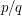and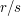are two distinct rational numbers written in their lowest terms, then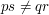, which implies that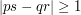, which implies that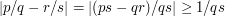. Therefore, ifis a real number and we can find a sequence of rational numbers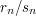(in their lowest terms, with denominators tending to infinity) such that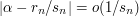(which is equivalent to saying that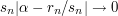), thencannot be rational. Loosely speaking, if you can approximatewell by rationals, thenis irrational. This turns out to be a very useful starting point for proofs of irrationality.

### Example 1

Let us construct inductively a sequence of rationals that approximate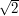. (This is not necessarily the best proof of the irrationality ofbut it gives an easy illustration of the technique.) We begin with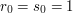, and observe that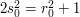. If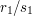were, then we would have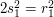, so the "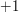" at the end of this is our error term in the first approximation. Now suppose we have defined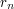and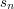in such a way that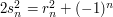. Then set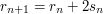and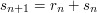. (The justification for this choice is that ifwerethen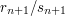would be too, as can easily be checked.) Then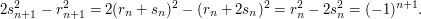Thus, we have constructed a sequence of rationals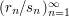, with denominators tending to infinity, such that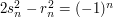for every. But from this we deduce that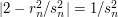, and therefore that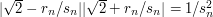. Since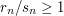(as may easily be checked), this implies that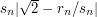tends to(at roughly the same rate as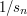), and therefore thatis irrational.

### Example 2

To prove the irrationality of, we start with the power-series expansion: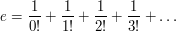We then setto be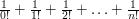. This is a fraction with denominatorthat divides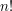. It differs fromby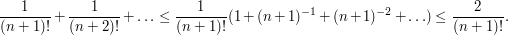Also, this difference is strictly positive and not zero. Therefore,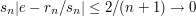, sois irrational.

### General discussion

The two proofs given so far can easily be, and usually are, presented in other ways that do not mention the basic principle explained in the quick description. However, sometimes that basic principle plays a much more important organizational role: one is given a numberto prove irrational, and one attempts to do so by finding a sequence of good rational approximations to.

Another point is that ifis irrational then such a sequence always exists: one can take the convergents from the continued-fraction expansion of. But this observation is less helpful than it seems, since for many important irrational numbers (such as) there does not seem to be a nice formula for the continued-fraction expansion. The point of the method explained here is that it is much more flexible: any sequence of good approximations will do (and sequences are indeed known that prove the irrationality of).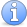Attention This article is in need of attention. It would be very nice to have a simple example of a number that has an explicit sequence of good rational approximations but for which there is no known formula for the continued-fraction expansion.

The following comments were made inline in the article. You can click on 'view commented text' to see precisely where they were made.

### One probably needs to insist

One probably needs to insist that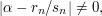to avoid the triviality of approximating a rational numberby the constant sequence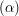. I'm not sure exactly how this is usually phrased in this context, so I will let someone else make the changes in the text.

### I was careful about that in

I was careful about that in the examples, but as you imply it is annoyingly difficult to find a good formulation for the quick description. I've gone for denominators tending to infinity when the fractions are written in their lowest terms. But in the case ofit is slightly easier to use the not-equal-to-formulation. If anyone has strong views about this, feel free to change it.

### Perhaps this could be

Perhaps this could be explicitly discussed in the article; not in the quick description, but perhaps further down, in the general discussion. One could explain (just as in your comment) that there are various slightly different approaches to avoiding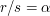: either by having a positive lower bound on the difference, or by havingtend to, staying coprime to.

Presumably at some point there will also be other articles on Diophantine approximation, including Liouville numbers and Roths' theorem, which will be linked to this article. Then one will be able to point out that even ifis already known to be irrational (so that in particular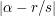is guaranteed to be positive), the question of bounding this quantity from above or below is still of great interest.

Another example that would be nice to include on this page eventually would be Apery's proof of irrationality of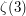. (I suspect that this has been suitably massaged by this stage that one could write down a pretty simple sequence of fractions that does the job.) I don't have the resources to hand at the moment to do this, but if I get a chance at some later point, I might add it. If someone else wants to beat me to it, please do!

The following comments were made inline in the article. You can click on 'view commented text' to see precisely where they were made.

### e+e^(-1) has sequence of

e+e^(-1) has sequence of approximations 2(1 + 1/2! + 1/4! + ... 1/(2n)!) and no immediately obvious (to me) formula for the continued-fraction expansion.

### Apery Constant

The use of this method for Zeta(3) is more involved but goes deeper into the principles of this method. I suggest that someone try to write up a description of this as Example 3.

## Post new comment

(Note: commenting is not possible on this snapshot.)

Before posting from this form, please consider whether it would be more appropriate to make an inline comment using the Turn commenting on link near the bottom of the window. (Simply click the link, move the cursor over the article, and click on the piece of text on which you want to comment.)